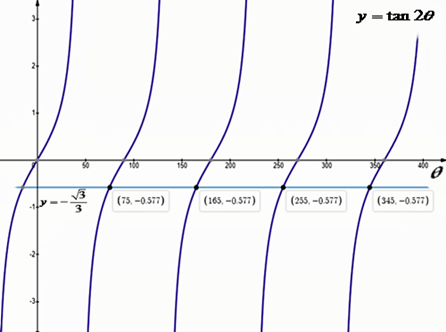# Find all solutions if 0^{circ} less than or equal to theta less than 360^{circ} . Verify...

## Question:

{eq}\tan 2 \theta = - \sqrt{3} / 3 {/eq}

## Trigonometric Equation:

In the treatment of the solution of a trigonometric equation, it is necessary to carry out the appropriate transformations that help us to simplify the trigonometric function as much as possible. Later, to isolate the variable {eq}\theta {/eq}, we use the corresponding inverse trigonometric function and then offer the solution in the indicated interval.

{eq}\eqalign{ & {\text{Let's solve the equation }}\,\tan 2\theta = - \frac{{\sqrt 3 }}{3}{\text{ for }}\,0 \leqslant \theta \leqslant 360^\circ {\text{. Then:}} \cr & {\text{We have that }}\,\tan \left( {150^\circ } \right) = - \frac{{\sqrt 3 }}{3} \cr & {\text{Using the inverse trigonometric function }}{\tan ^{ - 1}}\left( {\tan u} \right) = u{\text{ }} \cr & {\text{applying to both sides of the equation:}} \cr & \,\,\,\,\,\,\,{\tan ^{ - 1}}\left[ {\tan 2\theta } \right] = {\tan ^{ - 1}}\left[ { - \frac{{\sqrt 3 }}{3}} \right] \cr & {\text{We have that }}\,{\text{if }}\,\tan v = u\,\, \Rightarrow v = {\tan ^{ - 1}}\left( u \right){\text{, then solving for }}\,2\theta {\text{:}} \cr & \,\,\,\,\,\,\,2\theta = {\tan ^{ - 1}}\left( {\tan 2\theta } \right) = {\tan ^{ - 1}}\left( { - \frac{{\sqrt 3 }}{3}} \right) = 150^\circ \cr & {\text{The tangent function is periodic and is repeated every }}n \cdot 180^\circ ,{\text{ so:}} \cr & \,\,\,\,\,\,\,2\theta = 150^\circ + n \cdot 180^\circ ,\,\,\,{\text{ }}\,\,\left( {n = 0, \pm 1, \pm 2, \pm 3,...} \right) \cr & {\text{Solving for }}\,\theta {\text{:}} \cr & \,\,\,\,\,\,\,\theta = 75^\circ + n \cdot 90^\circ \cr & {\text{Since}}{\text{, the solutions for }}\,0 \leqslant \theta \leqslant 360^\circ {\text{ are:}} \cr & \,\,\,\,\,\,\,\boxed{\theta = 75^\circ ,\,\,\,\theta = 165^\circ ,\,\,\,\theta = 255^\circ \,{\text{ and }}\,\theta = 345^\circ } \cr} {/eq}